# Photoelectric effect facts for kids

Kids Encyclopedia Facts
 Photoelectric effect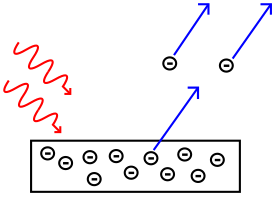A diagram that shows how electrons are emitted from a metal plate Light-matter interaction Low energy phenomena Photoelectric effect Mid-energy phenomena Compton scattering High energy phenomena Pair production

The photoelectric effect is a phenomenon in physics. The effect is based on the idea that electromagnetic radiation is made of a series of particles called photons. When a photon hits an electron on a metal surface, the electron can be emitted. The emitted electrons are called photoelectrons. The effect is also called the Hertz Effect, because it was discovered by Heinrich Rudolf Hertz, but this name is not used often. The photoelectric effect has helped physicists understand the quantum nature of light and electrons. The concept of wave–particle duality was developed because of the photoelectric effect. Albert Einstein proposed the Laws of Photoelectric Effect and won the Nobel Prize For Physics 1921.

## Mechanism

Not every electromagnetic wave will cause the photoelectric effect, only radiation of a certain frequency or higher will cause the effect. The minimum frequency needed is called the "cutoff frequency" or "threshold frequency'. The cutoff frequency is used to find the work function,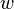$w$, which is the amount of energy holding the electron to the metal surface. The work function is a property of the metal and is not affected by the incoming radiation. If a frequency of light strikes the metal surface that is greater than the cutoff frequency, then the emitted electron will have some kinetic energy.

The energy of a photon causing the photoelectric effect is found through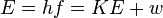$E = hf = KE + w$, where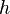$h$ is Planck's constant, 6.626×10−34 J·s,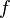$f$ is the frequency of the electromagnetic wave,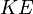$KE$ is the kinetic energy of the photoelectron and$w$ is the work function for the metal. If the photon has a lot of energy, Compton scattering (~ thousands of eV) or pair production (~ millions of eV) may take place.

The intensity of the light alone does not cause ejection of electrons. Only light of the cut off frequency or higher can do that. However, increasing the intensity of light will increase the number of electrons being emitted, as long as the frequency is above the cut off frequency.

## History

Heinrich Hertz made the first observation of the photoelectric effect in 1887. He reported that a spark jumped more readily between two charged spheres if light was shining on them. Further studies were done to learn about the effect observed by Hertz. In 1902, Philipp Lenard showed that the kinetic energy of a photoelectron does not depend on the light intensity. However, it was not until 1905 that Einstein proposed a theory that explained the effect fully. The theory says that electromagnetic radiation is a series of particles, called photons. The photons collide with the electrons on the surface and emit them. This theory ran against the belief that electromagnetic radiation was a wave. Thus, at first it was not recognised as correct. In 1916, Robert Millikan published the results of experiments using a vacuum photo-tube. His work showed that Einstein's photoelectric equation explained the behaviour very accurately. However, Millikan and other scientists were slower to accept Einstein's theory of light quanta. Maxwell's wave theory of electromagnetic radiation cannot explain the photoelectric effect and blackbody radiation. These are explained by quantum mechanics.

## Images for kidsPhotoelectric effect Facts for Kids. Kiddle Encyclopedia.